# NCERT solution for Class 6 Maths Chapter 13 Symmetry

In this page we have NCERT solution for Class 6 Maths Chapter 13:Symmetry . Hope you like them and do not forget to like , social share and comment at the end of the page.

## Exercise 13.1

Question 1
List any four symmetrical objects from your home or school.
(a)Paper sheet
(b) Kite
(c) CD
(d) Glass

Question 2
For the given figure, which one is the mirror line, l1 or l2?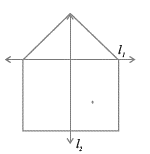l2

Question 3
Identify the shapes given below. Check whether they are symmetric or not. Draw the line of symmetry as well.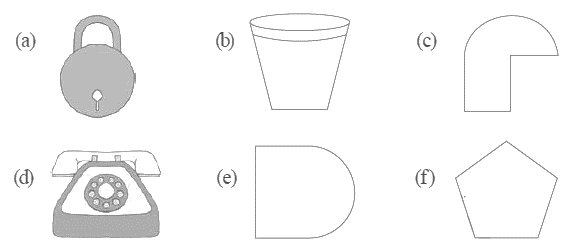(a) This is symmetrical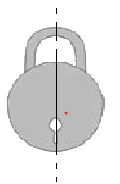(b) This is symmetrical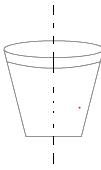(c) This is not symmetrical
(d) This is symmetrical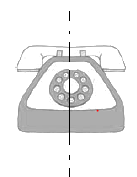(e) This is not symmetrical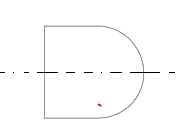(f) This is symmetrical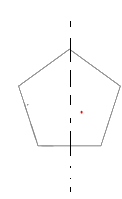Question 4
Copy the following on a squared paper. A square paper is what you would have used in your arithmetic notebook in earlier classes. Then complete them such that the dotted line is the line of symmetry.

Question 5
In the figure, l is the line of symmetry.
Complete the diagram to make it symmetric.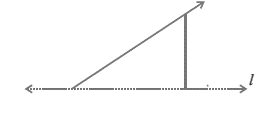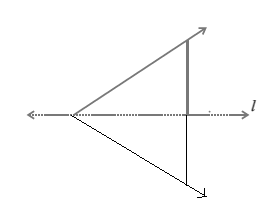Question 6
In the figure, l is the line of symmetry.
Draw the image of the triangle and complete the diagram so that it becomes symmetric.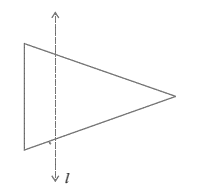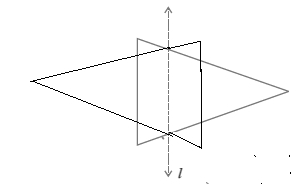## Exercise 13.2

Question 1
Find the number of lines of symmetry for each of the following shapes :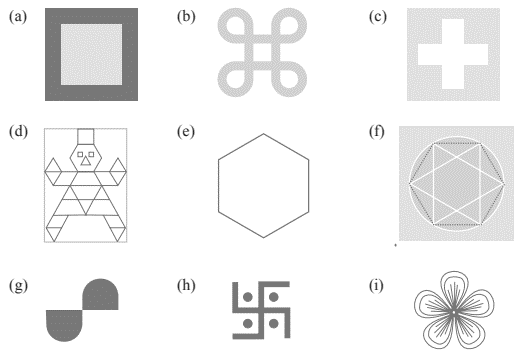(a) There are total 4 lines of symmetry in the given figure.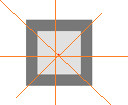(b) There are total 4 lines of symmetry in the given figure.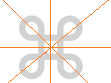(c) There are total 4 lines of symmetry in the given figure.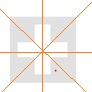(d) There is only 1 line of symmetry in the given figure.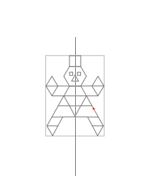(e) There are total 6 lines of symmetry in the given figure.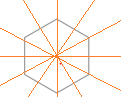(f) There are total 6 lines of symmetry in the given figure.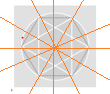(g) There is no line of symmetry in the given figure.
(h) There is no line of symmetry in the given figure.
(i) There are total 3 lines of symmetry in the given figure.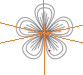Question 2
Copy the triangle in each of the following figures on squared paper. In each case, draw the line(s) of symmetry, if any and identify the type of triangle. (Some of you may like to trace the figures and try paper-folding first!)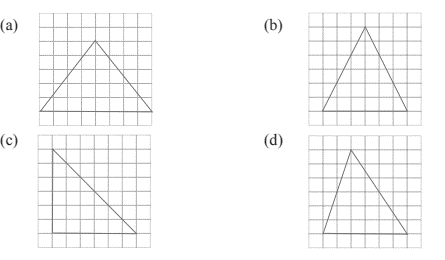(a) This figure has line of symmetry as L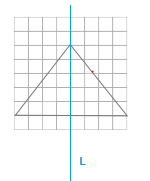(b) This figure has line of symmetry as M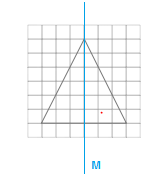(c)
This figure has line of symmetry as N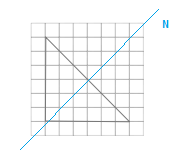(d) There is no line of symmetry

Question 3
Complete the following table.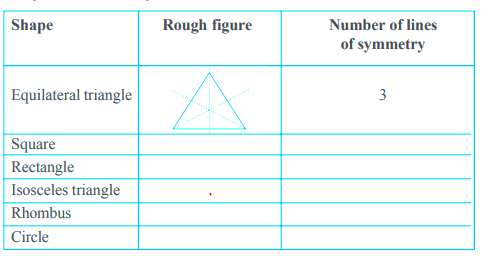(a) Square
Number of lines of symmetry:4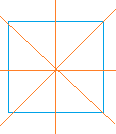(b) Square
Number of lines of symmetry:2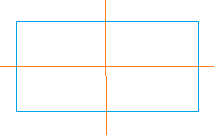(c) Isosceles Triangle
Number of lines of symmetry:1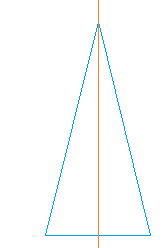(d) Rhombus
Number of lines of symmetry:2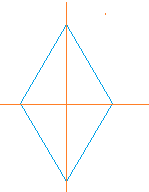(e) Circle
Number of lines of symmetry: infinite

Question 4. Can you draw a triangle which has
(a) exactly one line of symmetry?
(b) exactly two lines of symmetry?
(c) exactly three lines of symmetry?
(d) no lines of symmetry?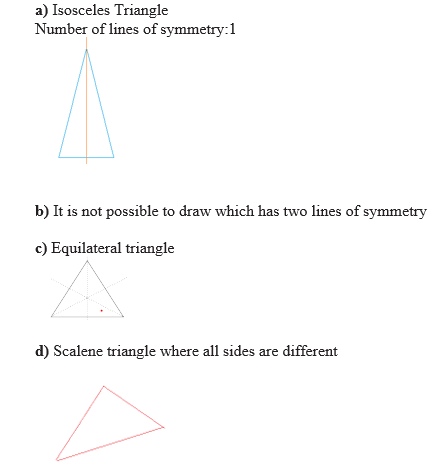Question 5
On a squared paper, sketch the following:
(a) A triangle with a horizontal line of symmetry but no vertical line of symmetry.
(b) A quadrilateral with both horizontal and vertical lines of symmetry.
(c) A quadrilateral with a horizontal line of symmetry but no vertical line of symmetry.
(d) A hexagon with exactly two lines of symmetry.
(e) A hexagon with six lines of symmetry.
(Hint : It will be helpful if you first draw the lines of symmetry and then complete the figures.)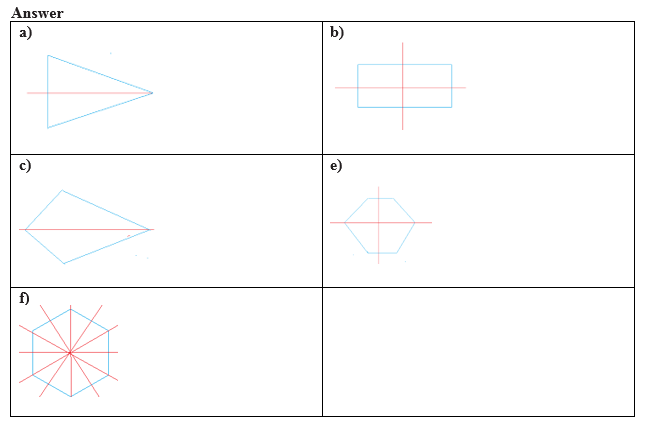Question 6
Trace each figure and draw the lines of symmetry, if any: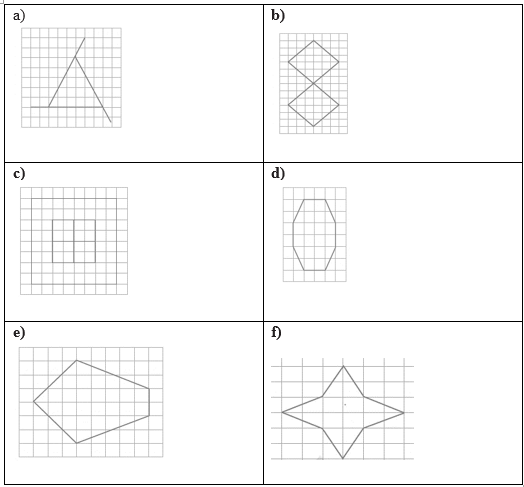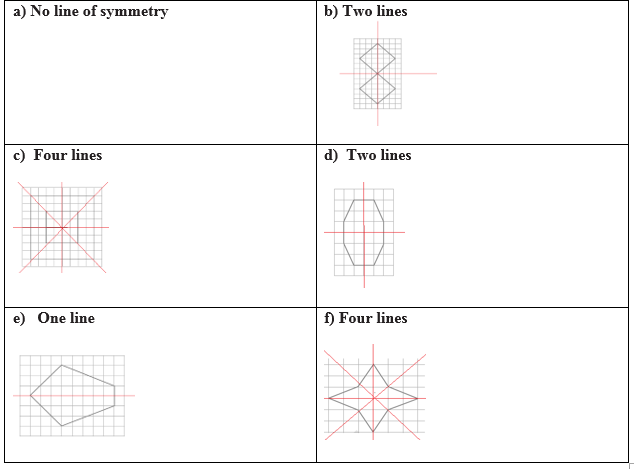Question 7

Consider the letters of English alphabets, A to Z. List among them
the letters which have
(a) vertical lines of symmetry (like A)
(b) horizontal lines of symmetry (like B)
(c) no lines of symmetry (like Q)
a) A,H,I,M,O,T,U,V,W,X,Y
b) B,C,D,E,H,I,K,O,X
c) F,G,J,L,N,P,Q,R,S,Z

Question 8
Given here are figures of a few folded sheets and designs drawn about the fold. In each case, draw a rough diagram of the complete figure that would be seen when the design is cut off

## Exercise 13.3

Question 1
Find the number of lines of symmetry in each of the following shapes. How will you check your answers?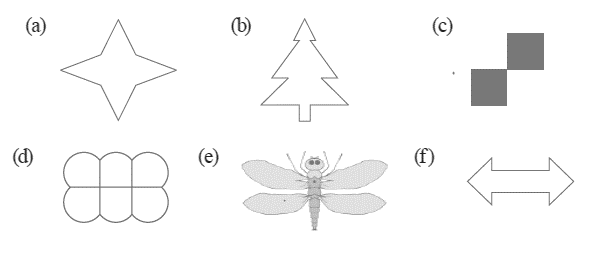Symmetry lines are shown by Blue lines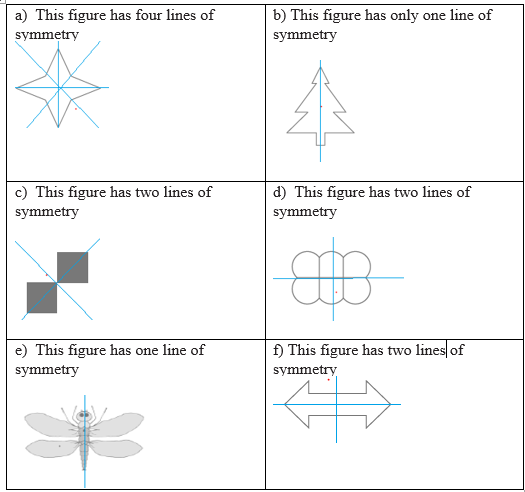Question 2
Copy the following drawing on squared paper. Complete each one of them such that the resulting figure has two dotted lines as two lines of symmetry.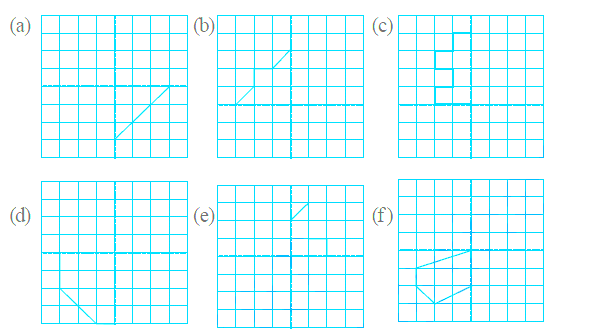(a)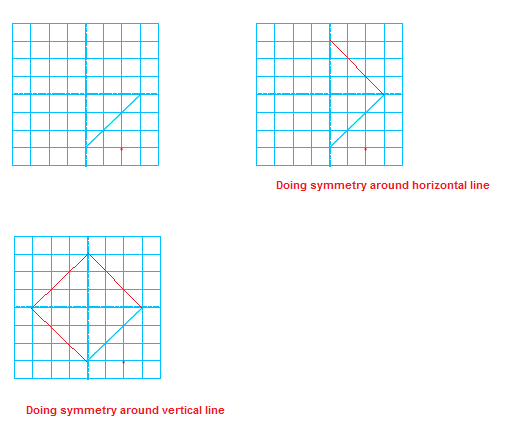(b)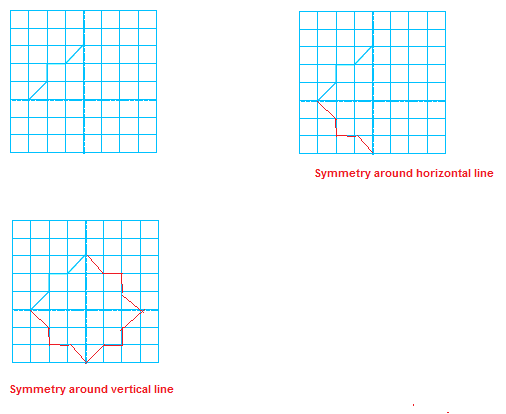(c)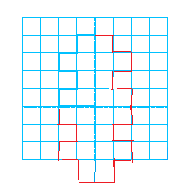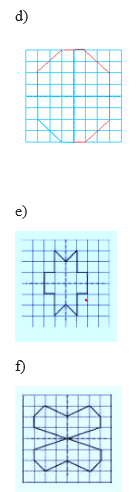Question 3
In each figure alongside, a letter of the alphabet is shown along with a vertical line. Take the mirror image of the letter in the given line. Find which letters look the same after reflection (i.e. which letters look the same in the image) and which do not. Can you guess why?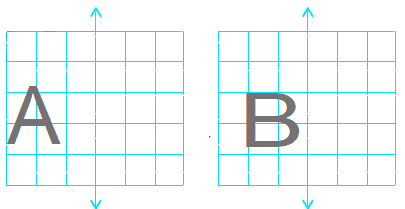Try for O E M N P H L T S V X
(a)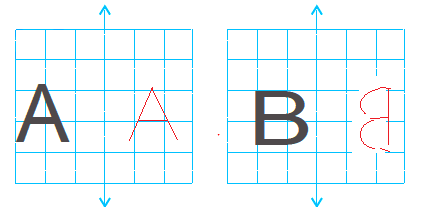(b)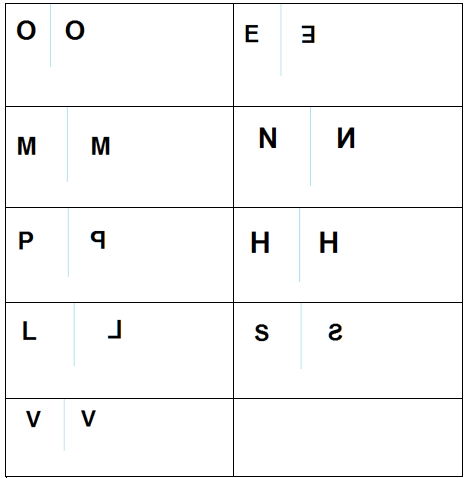• NCERT Solutions### Practice Question

Question 1 What is $\frac {1}{2} + \frac {3}{4}$ ?
A)$\frac {5}{4}$
B)$\frac {1}{4}$
C)$1$
D)$\frac {4}{5}$
Question 2 Pinhole camera produces an ?
A)An erect and small image
B)an Inverted and small image
C)An inverted and enlarged image
D)None of the above